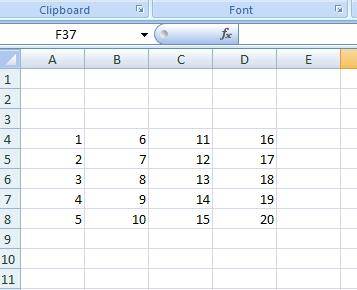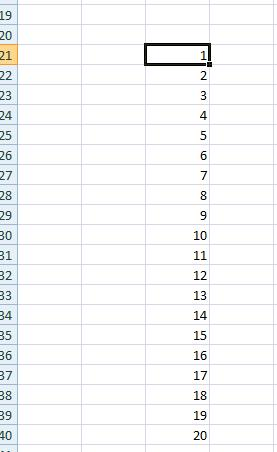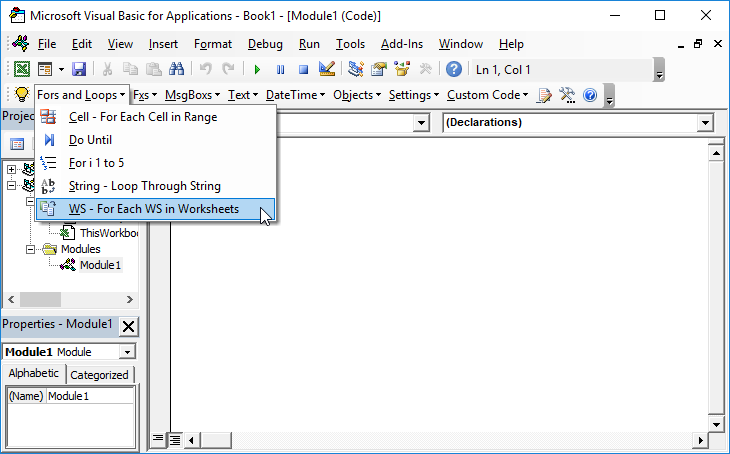# VBA – Convert Matrix to Vector

## Convert Matrix to a Vector

This is the opposite tutorial of the Converting a Single Row Vector into a Matrix tutorial.

The following function will take a range as a matrix and convert it to a single column vector:

``````Option Explicit
Function Create_Vector(Matrix_Range As Range) As Variant
Dim No_of_Cols As Integer, No_Of_Rows As Integer
Dim i As Integer
Dim j As Integer
Dim Cell
No_of_Cols = Matrix_Range.Columns.Count
No_Of_Rows = Matrix_Range.Rows.Count
ReDim Temp_Array(No_of_Cols * No_Of_Rows)
'Eliminate NULL Conditions
If Matrix_Range Is Nothing Then Exit Function
If No_of_Cols = 0 Then Exit Function
If No_Of_Rows = 0 Then Exit Function

For j = 1 To No_Of_Rows
For i = 0 To No_of_Cols - 1
Temp_Array((i * No_Of_Rows) + j) = Matrix_Range.Cells(j, i + 1)
Next i
Next j
Create_Vector = Temp_Array
End Function``````

So for example the vector:Will become:Where we have used the following subroutine to print out the vector:

``````Private Sub CommandButton1_Click()
Dim Vector
Dim k As Integer
Vector = Create_Vector(Sheets("Sheet1").Range("A4:D8"))
For k = 1 To UBound(Vector)
Sheets("Sheet1").Range("B20").Offset(k, 1).Value = Vector(k)
Next k
End Sub``````

Note that these routines work on both numbers and text.